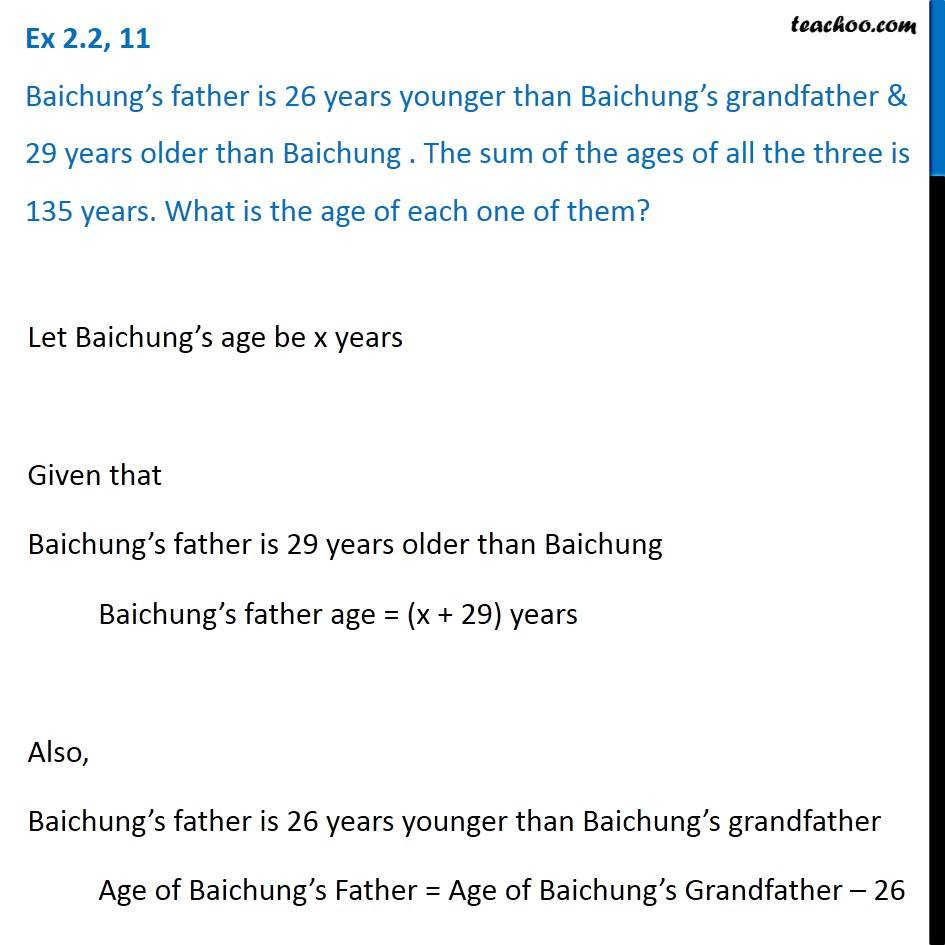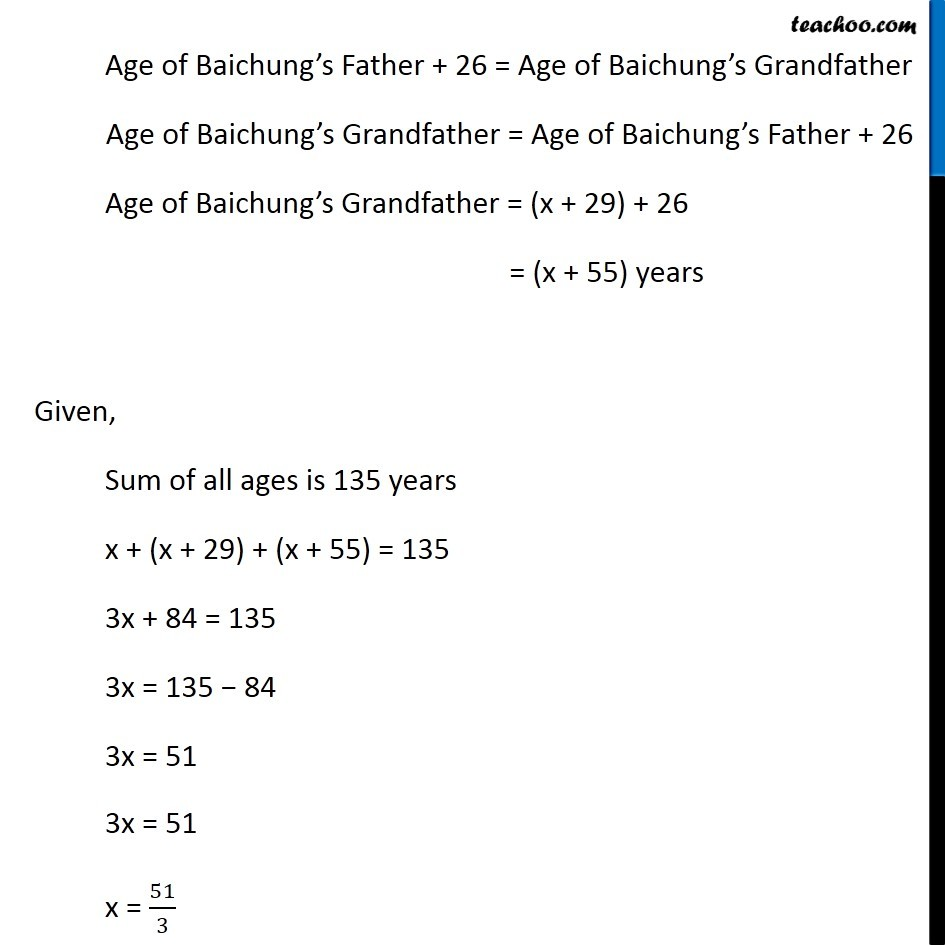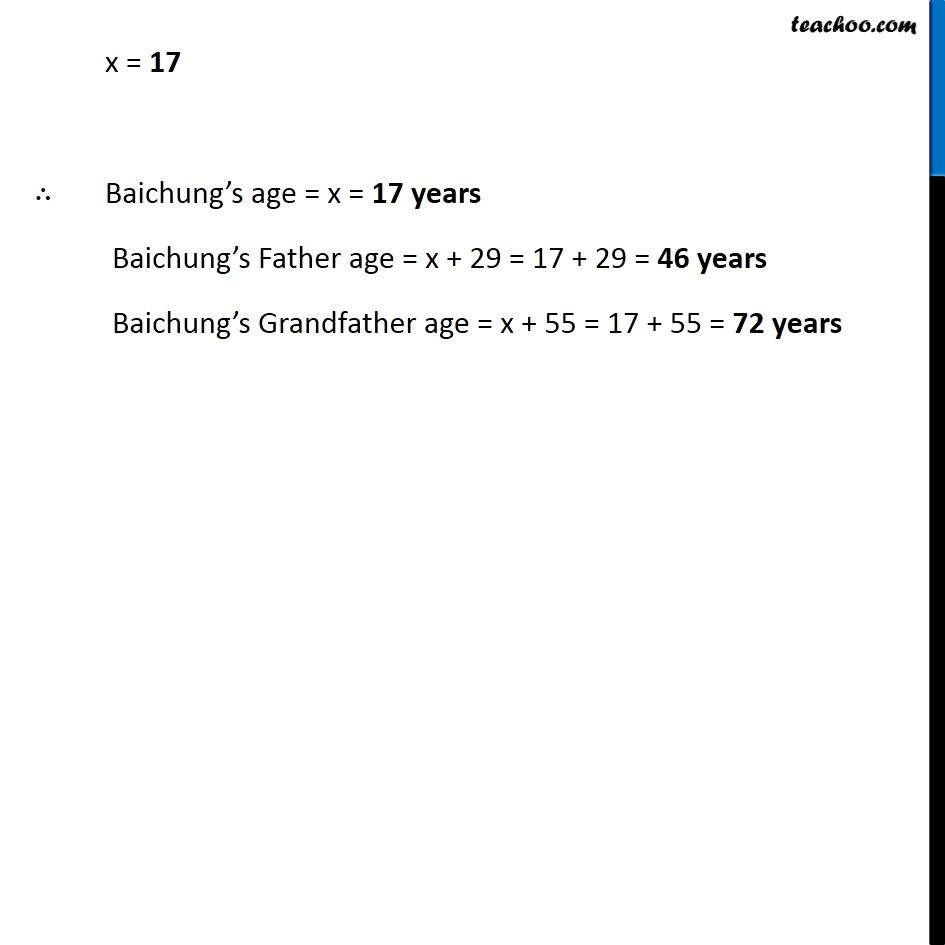Solving easy equations - Word Problems

Chapter 2 Class 8 Linear Equations in One Variable
Serial order wiseLearn in your speed, with individual attention - Teachoo Maths 1-on-1 Class

### Transcript

Question 11 Baichung’s father is 26 years younger than Baichung’s grandfather & 29 years older than Baichung . The sum of the ages of all the three is 135 years. What is the age of each one of them?Let Baichung’s age be x years Given that Baichung’s father is 29 years older than Baichung Baichung’s father age = (x + 29) years Also, Baichung’s father is 26 years younger than Baichung’s grandfather Age of Baichung’s Father = Age of Baichung’s Grandfather – 26 Age of Baichung’s Father + 26 = Age of Baichung’s Grandfather Age of Baichung’s Grandfather = Age of Baichung’s Father + 26 Age of Baichung’s Grandfather = (x + 29) + 26 = (x + 55) years Given, Sum of all ages is 135 years x + (x + 29) + (x + 55) = 135 3x + 84 = 135 3x = 135 − 84 3x = 51 3x = 51 x = 51/3 x = 17 ∴ Baichung’s age = x = 17 years Baichung’s Father age = x + 29 = 17 + 29 = 46 years Baichung’s Grandfather age = x + 55 = 17 + 55 = 72 years